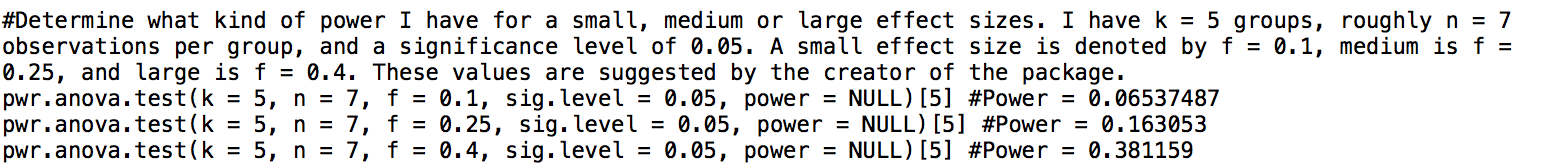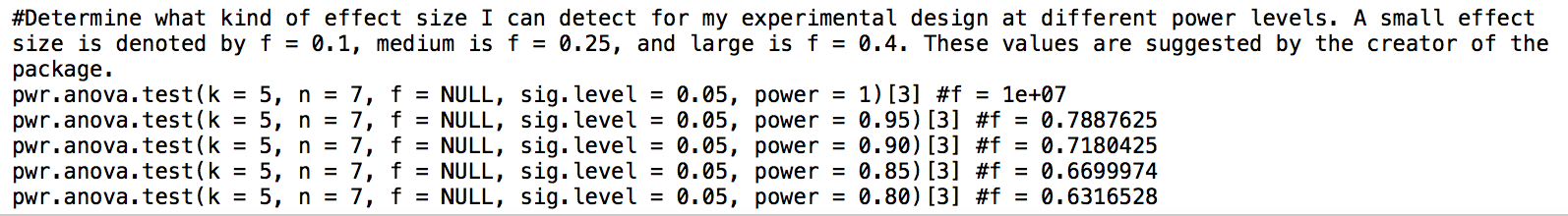# Remaining Analyses Part 5

## I’ve got the power

(Maybe).

I added a section to my boxplot R script for power analyses. I did two things: 1) calculate the power of my experimental design based on theoretical effect sizes and 2) calculated the detectable effect sized based on theoretical power values. To do this, I used the `pwr` R package.

Calculating power:Conclusion: I can detect a variety of effect sizes at very low power

Calculating effect size:Conclusion: If I have power, I can detect quite a bit

Written on November 13, 2017